COURSE
EXAM
STUDY MATERIALS

Click Question to Get Free Answers >

Areas Of Parallelograms And Triangles NCERT Solutions : Class 9 Maths

NCERT Solutions for Class 9 Maths : Areas Of Parallelograms And TrianglesFilter
Filters :
Classes
•
•
•
•
•
•
•
Chapters
•
•
•
•
•
•
•
•
•
•
• 6 More
Exercises
•
•
•
•
•

Doubtnut one of the best online education platform provides free NCERT Solutions of Maths for Class 9 Areas Of Parallelograms And Triangles which are solved by our maths experts as per the NCERT (CBSE) guidelines. We provide the solutions as video solutions in which concepts are also explained along with answer which will help students while learning , preparing for exams and in doing homework. These Solutions will help to revise complete syllabus and score more marks in examinations.

Get here free, accurate and comprehensive NCERT Solutions for Class 9 Maths Areas Of Parallelograms And Triangles which have been reviewed by our maths counsellors as per the latest edition following up the CBSE guidelines. We provide video solutions in which solutions to all the questions of NCERT Class 9 Maths textbook are explained in step by step and detailed way.

The Topics of the Chapter Areas Of Parallelograms And Triangles are :

PARALLELOGRAM

INTRODUCTION

PARALLELOGRAM ON THE SAME BASE AND BETWEEN THE SAME PARALLELS

TRIANGLE ON THE SAME BASE AND BETWEEN THE SAME PARALLELS

CONGRUENCE

It contains these exercises along with solved examples. All the exercises are solved in the video. Select the exercise to view the solutions exercisewise of the Chapter Areas Of Parallelograms And Triangles:

NCERT Solutions Class 9 Maths Chapter Areas Of Parallelograms And Triangles Exercises:

We have covered all the exercises and also Solved examples in the videos. Along with the practise exercise Students should also practise solved examples to clear the concepts of the Areas Of Parallelograms And Triangles. If incase you have any doubt you can watch the solutions for the given questions.Watch the solutions for the given questions in which it is also explained in the video steps to solve the questions along with answers.

The NCERT book provides the perfect explanation of the topics and subtopics related to the chapter in the appropriate manner. It describes the concept in the most accessible and easy to understand way for the benefit of the students in a brief yet comprehensive manner. Every concept has a detailed explanation, which will help the students to solve the problems of class 9 maths, and the best part is, it is put in such a systematic manner that it won’t increase the length of the chapter which is the fearsome thing for a student. The precise the solutions of the chapter, the more student is willing to read. NCERT books have not left any topic untouched and explained the appropriate amount the much needed for the child to know of that age. It is best to read the NCERT books above all because of its authenticity.

Especially for the students of CBSE board, the NCERT books should be prioritized. There are numerous amount of authors and publications, but what makes NCERT books different is that it made sure that the needs of regular children are addressed. They focus on the process of learning than just the outcome or results. The physical aspect is another plus point, it is printed at color, least price for the accessibility of student of every background they work on ‘no-profit-no-loss’ concept which is beneficial for the education system.

One can score the best in their boards by being thorough with each and every concept of NCERT books. Considering all the stuff provided in the content including all the theory, in text questions and exercise questions. When you are able to answer all of them, you can crack the board without any problem. Even one can qualify JEE, NEET, and all those examinations by sticking to the core content of NCERT, one paragraph of NCERT books covers one page of any other reference book.

One should go through the book daily. It will help them understand the methodologies and formulas in a better way than just going and doing the problems once.

We at Doubtnut provide an in-depth, detailed and easy to understand solutions to all the questions for NCERT and also provide the pdf format of the book, which is easily accessible by everyone. We ensure that solutions are comprehensive and every step is clearly mentioned. Solutions are focused on learning various Mathematics tricks and shortcuts for quick and easy calculations. These solutions will change your approach towards studies and will make you realize how interesting this subject is.

Area of Parallelograms and Triangles – Class 9 Maths NCERT Solutions

The chapter as the name suggests deals with the two major shapes called parallelogram and triangles.

Area of parallelogram = base (B) × Perpendicular height (h)

Area of triangle= base*height/2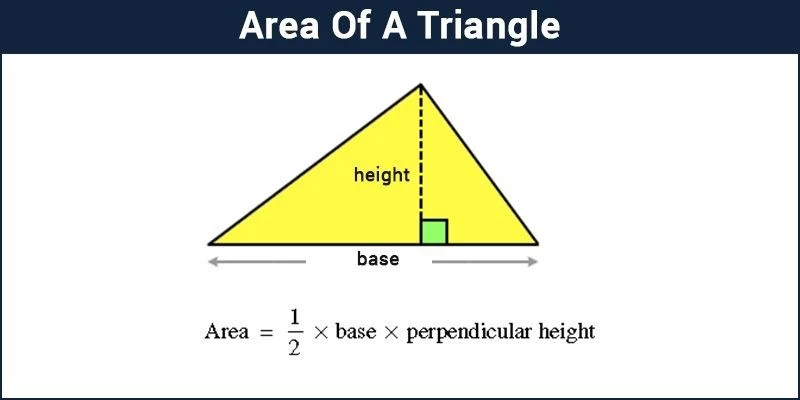DEFINITION:

PARALLELOGRAM

A flat shape with four straight sides. The opposite sides are equal and parallel to each other.TRIANGLE

A shape that has three straight lines.

UNIT: SI UNIT: meter ²

CGS UNIT: centimeter ²

FIGURES ON THE SAME BASE AND BETWEEN THE SAME PARALLELS

EXAMPLE: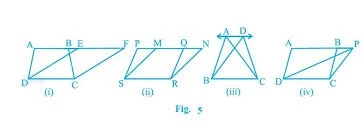Figures are said to be on the same base and between same parallels if they are sharing the common base and their opposite sides are parallel to each other.

In the given fig: 6.44 DBC and BDA shares the same base that is BI and lies between the same parallel that is CD and AB, hence through this concept it’s is found that

Area of (ABC) ∕ Area of (DBC) =AO ∕ DO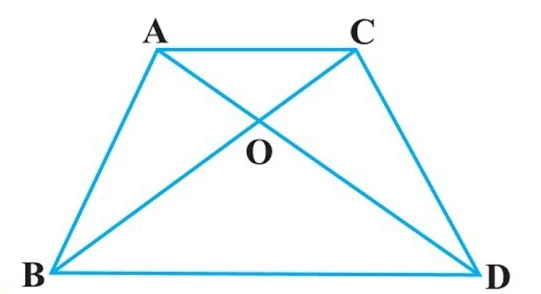EXAMPLE: If a triangle and a parallelogram are on the same base and between same parallels, then prove that the area of a triangle is equal to half the area of a parallelogram.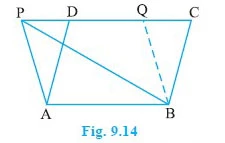SOL: let ABP and parallelogram ABCD are on the same base AB and between the same parallel AB and PC (fig 9.14)

We wish to prove that area (PAB) =½ area (ABCD)

Drawing BQ ‖ AP to obtain another parallelogram ABQP. Now parallelograms ABQP and ABCD are on the same base AB and between same parallel AB and PC.

So, two figures are said to be on the same base and between same parallels, if they have a common base (sides) and vertices (or the vert*) opposite to the common base if each figure lies on a line parallel to the base.

PARALLELOGRAMS ON THE SAME BASE AND BETWEEN THE SAME PARALLELS:

EXAMPLE: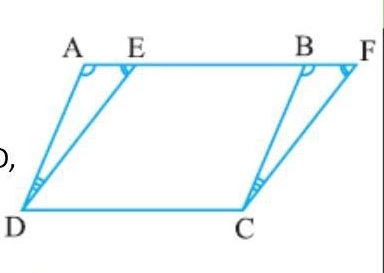In the given figure we can see that the parallelogram ABCD and EFCD share the same base that is DC and lies between the same parallels that are AF and CD.

THEOREM:

The parallelograms sharing the same base and lies between the same parallels are equal.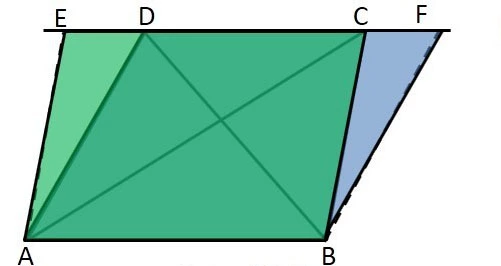In ABFD, DF ‖ AD              (given as AB‖CD)  and BF‖AD          (by construction)      Thus, both pairs of opposite sides are parallel.    Hence, ABFD is a parallelogram. In ABCE, EC ‖ AB            (given as AB‖CD) and AE‖BC        (by construction) Thus, both pairs of opposite pairs are parallels. Hence, ABCE is a parallelogram.

Thus, ABFD and ABCE are two parallelograms with same base AB and between two parallels AB and EF.

→ Area (ABFD) = area (ABCE)    (the area between same parallels and on the same base are equal)

TWO TRIANGLES ON THE SAME BASE AND BETWEEN THE SAME PARALLELS

EXAMPLE: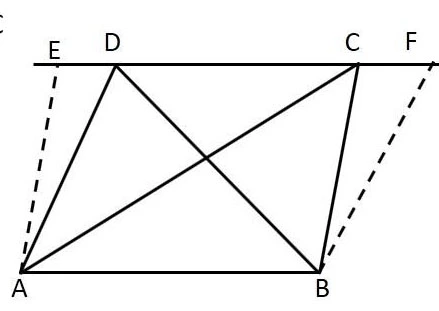ABD and ABC are triangles on the same base that is AB and between same parallels lines that is AB and DC.

THEOREM:

Two triangles on the same base or sharing the same base and between the same parallel lines are equal.

Given: ADB and ABC are a triangle on the base AB and between the same parallels AB and DCProve: area (ADB) =area (ABC)

Construction:

Construct a line through A parallel to BC meeting DC at E that is AE‖BC and construct a line through B parallel to AD meeting DC at F that is BF‖AD.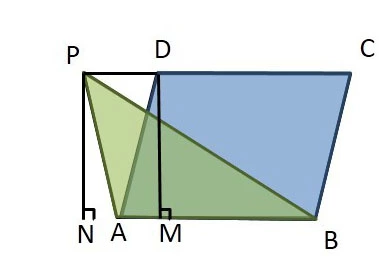In other words, the two triangles lying on the same base and between the same parallels are equal in areas.

The relation between the area of a triangle and the area of a parallelogram:

Given: A parallelogram ABCD and ABP on the same base AB and between the same parallels.

To Prove: area of a triangle is equal to half the area of parallelogram area (ABP) = ½ area (ABCD)

Construction: Join DP

Let DM AB and PN AB

Proof: area of the triangle ABP is half the area of parallelogram ABCD.

AREA OF A PARALLELOGRAM

Area of a parallelogram is the product of base and perpendicular dropped on that base from its opposite vert*.

Area of parallelogram =base× perpendicular height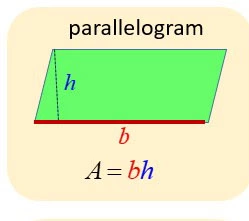AREA OF A TRIANGLE

Area of a triangle is the product of the perpendicular height of the triangle and base divided by 2(half the product of base and height

Area of triangle=½(base × perpendicular height)

EXAMPLES: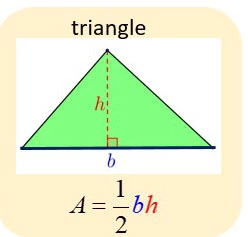Question: P and Q are any point lying on the side DC and AD, respectively of a parallelogram ABCD shows that ar(APB) = ar(BQC)

Solution: given. A parallelogram ABCD .P and Q are any two points lying on the sides DC and AD, respectively.

Now, parallelograms ABCD and BQC stand on the same base BC and lie between the same parallel lines BC and AD.

Therefore (BQC) = ½ area (ABCD)           1

Similarly APB and parallelogram ABCD stands on the same base AB and lie between the same parallel AB and CD

Therefore area (APB) = ½area (ABCD)        2

From equation (1) and (2) we get,

Area (APB) =area (BQC)

EXAMPLE:

How to find the area of the following triangle?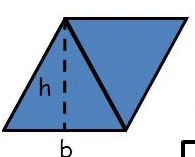SOLUTION:

Area of a parallelogram is equal to b*h

Two triangles make the parallelogram

Area of one triangle is equal to ½area of a parallelogram

Area of the triangle is equal to ½(b×h).

RULES TO DO THE QUESTIONS OF AREA OF PARALLELOGRAM AND TRIANGLES

1. Identifying figures lying on the same base and between the same parallels.

2. Relate the areas of the figures lying between the same base and between the same parallels.

SOME IMPORTANT POINTS:

A parallelogram is a quadrilateral that is it is made up of 4 lines segments in which the opposite sides are parallel and equal.

EXAMPLES of quadrilaterals are – a rectangle, a square, a rhombus, and they are also a special type of parallelograms.

Properties of a parallelogram are-

1. Area of a parallelogram is equal to base × altitude.

2. Sum of four angles of a parallelogram is 360 degrees.

3. Diagonals of a parallelogram bisect each other.

4. The sum of adjacent angles of a parallelogram is 180 degrees.

FOR A QUADRILATERAL THESE FOUR STATEMENTS ARE EQUIVALENT

1. Diagonals bisect one another.

2. Opposite sides are parallel.

3. Opposite angles are equal.

4. Opposite sides are equal.

Significance of NCERT Mathematics Book for Class 9

NCERT Mathematics book for Class 9 makes the transition to the class easier for students and students don’t feel that difficult to adapt to the new difficulties. The concepts are explained in a very easy to understand manner and a huge question bank is provided to practice questions and grasp concepts to depth. Learning from this book makes learning processes easy and students easily cope with the class.

Some important features of the book are:

• Easy to understand and detailed explanation of concepts.
• A huge question bank to practice questions from.
• Notes, formulas and important things are given at the end of every chapter.
• Aided with online solutions.
• Strictly follow the latest CBSE guidelines and syllabus.

NCERT is the best book available in the market to get better at maths and achieve high scores in exams. It provides everything from well-explained theories to a variety of problems to practice from. It makes the learning process very easy. In addition, whatever is left in the book is covered by our online educational learning platform, Doubtnut in the form of video tutorials, which makes it fun for students to learn the subject.